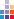# Program to check whether given two numbers are Amicable or not

Amicable numbers are pair of those two numbers so related that sum of the proper divisors of the one is equal to the other for example (220,284). Propser divisors of 220 are 1,2,4,5,10,11,20,22,44,55, and 110 and the sum of these is 284; and proper divisors of 284 are 1,2,4,71, and 142 and sum of these numbers is 220

 1 `/*Program to check whether given two numbers are Amicable or not*/` 2 `#include ` 3 `#include ` 4 `void main()` 5 `{` 6 ` int num1,num2,i,sum_n1=0,sum_n2=0;` 7 8 ` printf("****Programme to check whether given two numbers are Amicable or not****");` 9 ` ` 10 ` //Take two numbers from user` 11 ` printf("Enter Number1: ");` 12 ` scanf("%d",&num1);` 13 ` printf("Enter Number2: ");` 14 ` scanf("%d",&num2);` 15 16 ` //Calculate the sum of proper divisors of num1` 17 ` printf("%d --> ",num1);` 18 ` for(i=1; i<=num1/2; i++)` 19 ` {` 20 ` if(num1%i==0)` 21 ` {` 22 ` printf("%d + ",i);` 23 ` sum_n1=sum_n1+i;` 24 ` }` 25 ` }` 26 ` printf(" = %d",sum_n1);` 27 ` printf("");` 28 29 ` //Calculate the sum of proper divisors of num2` 30 ` printf("%d --> ",num2);` 31 ` for(i=1; i<=num2/2; i++)` 32 ` {` 33 ` if(num2%i==0)` 34 ` {` 35 ` printf("%d + ",i);` 36 ` sum_n2=sum_n2+i;` 37 ` }` 38 ` }` 39 ` printf(" = %d",sum_n2);` 40 41 ` //Check the sum of num1's divisors and num2's divisors` 42 ` if(sum_n1==num2 && sum_n2==num1)` 43 ` {` 44 ` printf("Yes Amicable Number");` 45 ` }` 46 ` else` 47 ` {` 48 ` printf("No Not Amicable Numbers");` 49 ` }` 50 ` ` 51 52 ` getch();` 53 `}`by Seven on 16-Aug-2015 10:53 pm
Can be optimized using i<=(num1/2) in for loop
•  4 Like
•  3 Dislike
• Reportby Sachin Puri on 23-Dec-2015 12:59 am
Thanks
•  3 Like
•  3 Dislike
• Reportby Manish on 26-Jun-2012 12:33 pm
nice......
•  3 Like
•  4 Dislike
• Reportby rohan on 12-Feb-2011 10:56 am
gud job sir, lz sir if ucan explain for(i=1; i
•  6 Like
•  6 Dislike
• Reportby Manoj Srivastav on 09-Apr-2011 01:47 am
for loop is being used here to iterate through all the numbers which are less then num1 or num2 and check whether they are proper divisor or not.Hi, I am Sachin Puri, I am a Programmer. Programming is my work and it is the thing i like to do most in my free time.